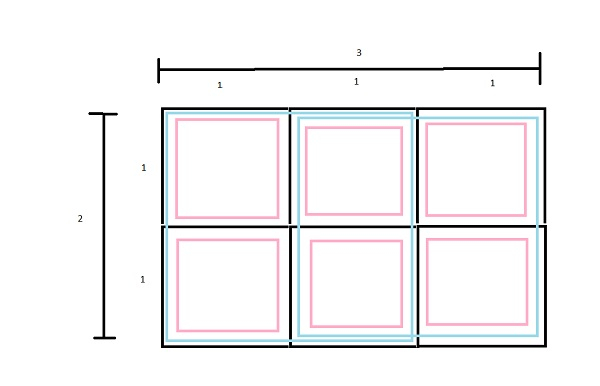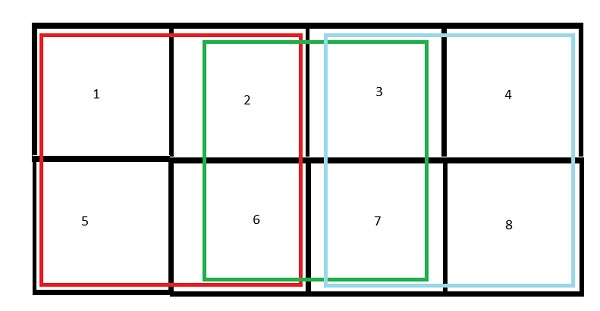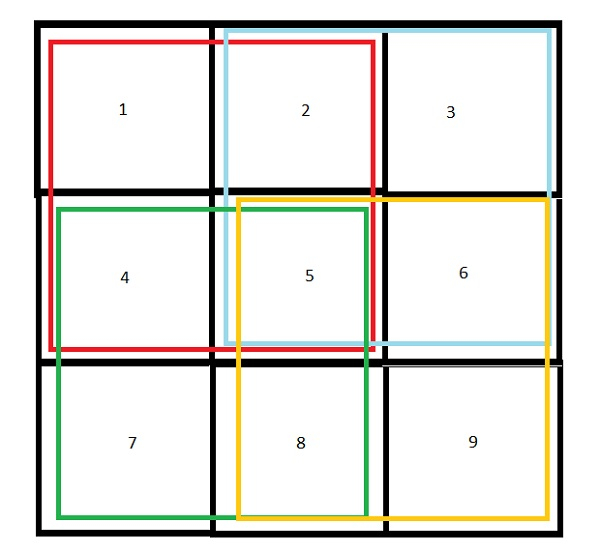# Count number of squares in a rectangle in C++

We are given with a rectangle of length L and breadth B, such that L>=B. The goal is to find the number of squares that a rectangle of size LXB can accommodate.Above figure shows a rectangle of size 3 X 2. It has 2, 2X2 squares and 6,1X1 squares in it.

Total squares= 6+2=8.

• Every rectangle of size LXB has L*B number of 1X1 squares.

• Biggest squares are of size BXB.

• For L=B=1, squares = 1.

• For L=B=2, squares = 1 + 4 = 5. ( 1 of 2X2, 4 of 1X1 )

• For L=B=3, squares = 1 + 4 + 9 = 14. ( 1 of 3X3, 4 of 2X2, 9 of 1X1 )

• For L=B=4, squares = 1 + 4 + 9 + 16 = 30 ( 1 of 4X4, 4 of 3X3, 9 of 2X2, 16 of 1X1 )

• ……………..

• For every BXB rectangle number of squares is

for ( i=1 to B )
No. of squares + = i*i.

When L>B. More squares will be added. When L=B+1 ( 1 extra column than B ). Then squares added will be L + ( L-1) + ….+3+2+1 = L(L+1)/2

So for extra L-B columns squares added will be ( L-B ) * (B)(B+1)/2

• Total squares will be squares in BXB + (L-B) * (L)(L+1)/2.

• You can also use formula B(B+1)(2B+1)/6 for the series (1 + 4 + 9 +......BXB) in step 8.

Let’s understand with examples.

Input − L=4, B=2

Output − Count of squares in rectangle − 11

Explanation − 8 squares of 1X1 and 3 of 2X2.Input − L=3, B=3

Output − Count of squares in rectangle − 14

Explanation − 9 squares of 1X1 , 4 of 2X2 and 1 of 3X3.## Approach used in the below program is as follows

• We take an integers length and breadth for dimensions of rectangle.

• Function numofSquares(int l, int b) takes dimensions and returns the number of squares in the rectangle of size lXb.

• For biggest squares bXb. Use for loop from 1 to b and add each i*i to squares.

• Now if l>b. New added squares will be (l-b)(b)(b+1)/2. Add this to squares.

• Return squares as desired result.

## Example

Live Demo

#include<iostream>
using namespace std;
int numofSquares(int l, int b){
int squares=0;
squares += i*i;
}
squares+=(l-b)*b*(b+1)/2;
return squares;
}
int main(){
int length = 5, breadth = 4; //keep length always >= breadth
cout << "Count of squares is :"<< numofSquares(length,breadth);
}

## Output

If we run the above code it will generate the following output −

Count of squares is :40